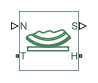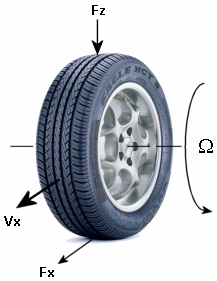Tire-road dynamics given by Magic Formula coefficients

•Libraries:
Simscape / Driveline / Tires & Vehicles / Tire Subcomponents

## Description

The Tire-Road Interaction (Magic Formula) block represents the interaction between the tire tread and the road pavement. The Magic Formula predicts the longitudinal force that results from this interaction by using an empirical equation based on fitting coefficients. The block ignores tire properties such as compliance and inertia.

The block represents the longitudinal forces at the tire-road contact patch using the Magic Formula of Pacejka .

The figure displays the forces on the tire. The table defines the tire model variables.SymbolDescription
ΩWheel angular velocity.
VxWheel hub longitudinal velocity.
${r}_{w}\Omega$Tire tread longitudinal velocity.
uTire longitudinal deformation.
${V}_{T}={r}_{w}{\Omega }^{\prime }={r}_{w}\Omega +\stackrel{˙}{u}$Tire tread longitudinal velocity. Typically, the tire tread longitudinal velocity has a component due to tire rotation, rwΩ, and an optional component due to tire deformation, $\stackrel{˙}{u}$. Because port T computes VT, the calculations for tire rotations and deformation occur outside of the block.
${V}_{sx}={V}_{x}-{V}_{T}$Contact patch slip velocity. If there is no tire longitudinal compliance, then $u=0$.
$k=-\frac{{V}_{sx}}{{|{V}_{x}|}_{smooth}}$Wheel slip for a tire without compliance.
${F}_{x}=f\left(k,{F}_{z}\right)$Longitudinal force exerted on the tire at the contact point.
f is a characteristic function of the tire.

### Tire Response

You can model tire roll and slip.

Forces and Characteristic Function

The block uses a steady-state tire characteristic function, ${F}_{x}=f\left(k,{F}_{z}\right)$, where:

• Fx is the longitudinal force on the tire.

• Fz is the vertical load.

• k is the wheel slip

Roll and Slip

The equation for translational motion of a non-slipping, non-compliant tire is ${V}_{x}={r}_{w}\Omega$. When tires experience slip, they develop a longitudinal force, Fx.

The contact patch slip velocity is ${V}_{sx}={V}_{x}-{r}_{w}\Omega -\stackrel{˙}{u}$. For a tire without compliance, u = 0. The unsmoothed contact patch slip is

`$k=-\frac{{V}_{sx}}{|{V}_{x}|},$`

and the block saturates the slip denominator as

where VXLOW is the Lower boundary of slip denominator, VXLOW parameter. The block smoothly transitions |Vx| to VXLOW over the transition regions -VXLOW - Vth/2 < Vx < -VXLOW + Vth/2 and VXLOW - Vth/2 < Vx < VXLOW + Vth/2. The block saturates slip according to

where kpumin is the Minimum valid wheel slip, KPUMIN parameter and kpumax is the Maximum valid wheel slip, KPUMAX parameter. The block transitions k over the regions kpumin - kth/2 < k < kpumin + kth/2 and kpumax - kth/2 < k < kpumax + kth/2. The block defines the slip smoothing threshold as

`${k}_{th}=\frac{{V}_{th}}{1m/s}.$`

For this equation, a locked, sliding wheel has k = -1. For perfect rolling, k = 0.

Magic Formula Coefficients for Typical Road Conditions

The block uses numerical values based on empirical tire data. These values are typical sets of constant Magic Formula coefficients for common road conditions.

SurfaceBCDE
Dry tarmac101.910.97
Wet tarmac122.30.821
Snow520.31
Ice420.11

### Parameterizations

Peak Longitudinal Force and Corresponding Slip

When you set Parameterize by to ```Peak longitudinal force and corresponding slip```, the block uses a representative set of Magic Formula coefficients. The block scales the coefficients to yield the peak longitudinal force Fx0 at the corresponding slip κ0 that you specify, for rated vertical load Fz0.

Magic Formula with Constant Coefficients

When you set Parameterize by to ```Constant Magic Formula coefficients```, the block uses the dimensionless coeffiecients, B, C, D, and E, or stiffness, shape, peak, and curvature, such that

`${F}_{x}=f\left(\kappa ,{F}_{z}\right)={F}_{z}\cdot D\cdot \mathrm{sin}\left(C\cdot \mathrm{arctan}\left\{B\kappa -E\left[B\kappa -\mathrm{arctan}\left(B\kappa \right)\right]\right\}\right).$`

The slope of f at $k=0$ is $BCD\cdot {F}_{z}$.

When you set Parameterize by to `Load-dependent Magic Formula coefficients`, the block uses dimensionless coefficients that are functions of the tire load. A more complex set of parameters `p_i`, entered in the property inspector, specifies these functions:

`${F}_{x0}={D}_{x}\mathrm{sin}\left({C}_{x}\mathrm{arctan}\left\{{B}_{x}{\kappa }_{x}-{E}_{x}\left[{B}_{x}{\kappa }_{x}-\mathrm{arctan}\left({B}_{x}{\kappa }_{x}\right)\right]\right\}\right)+{S}_{Vx},$`

where:

`${\kappa }_{{}_{x}}=\kappa +{S}_{{}_{Hx}}$`

`${D}_{\text{x}}={\mu }_{\text{x}}·{F}_{\text{z}}$`

`${B}_{\text{x}}=\frac{{K}_{\text{x}\kappa }}{{C}_{\text{x}}{D}_{\text{x}}+{\epsilon }_{\text{x}}}$`

SHx and SVx represent offsets to the slip and longitudinal force in the force-slip function, or horizontal and vertical offsets if the function is plotted as a curve. μx is the longitudinal load-dependent friction coefficient. εx is a small number that prevents division by zero as Fz approaches zero.

### Assumptions and Limitations

The Tire-Road Interaction (Magic Formula) block assumes only longitudinal motion and includes no camber, turning, or lateral motion.

## Ports

### Input

expand all

Physical signal input port associated with the normal force acting on the tire, in N. The normal force is positive if it acts downward on the tire, pressing it against the pavement.

Physical signal input port associated with the Magic Formula coefficients. Provide the Magic Formula coefficients as a four-element vector specified in the order [B, C, D, E].

#### Dependencies

To enable this port, set Parameterize by to ```Physical signal Magic Formula coefficients```.

### Output

expand all

Physical signal output port associated with the relative slip, k, between the tire and road.

### Conserving

expand all

Mechanical rotational port associated with the tire tread.

Mechanical translational port associated with the wheel hub that transmits the thrust generated by the tire to the remainder of the vehicle.

## Parameters

expand all

### Main

Magic Formula tire simulation option. Select how the block parameterizes the tire using the Magic Formula:

• ```Peak longitudinal force and corresponding slip``` — Parameterize the Magic Formula by using the physical characteristics of the tire.

• ```Constant Magic Formula coefficients``` — Specify the parameters that define the constant B, C, D, and E coefficients as scalars.

• ```Load-dependent Magic Formula coefficients``` — Specify the parameters that define the load-dependent C, D, E, K, H, and V coefficients as vectors, one for each coefficient.

• ```Physical signal Magic Formula coefficients``` — Specify the Magic Formula coefficients using port M as a four-element vector in the order [B, C, D,E].

#### Dependencies

To enable this parameter, set Parameterize by to ```Peak longitudinal force and corresponding slip```.

Maximum longitudinal force Fx0 that the tire exerts on the wheel when the vertical load equals its rated value Fz0.

#### Dependencies

To enable this parameter, set Parameterize by to ```Peak longitudinal force and corresponding slip```.

Contact slip κ'0, expressed as a percentage (%), when the longitudinal force equals its maximum value Fx0 and the vertical load equals its rated value Fz0.

#### Dependencies

To enable this parameter, set Parameterize by to ```Peak longitudinal force and corresponding slip```.

#### Dependencies

To enable this parameter, set Parameterize by to ```Constant Magic Formula coefficients```.

#### Dependencies

To enable this parameter, set Parameterize by to ```Constant Magic Formula coefficients```.

#### Dependencies

To enable this parameter, set Parameterize by to ```Constant Magic Formula coefficients```.

#### Dependencies

To enable this parameter, set Parameterize by to ```Constant Magic Formula coefficients```.

Nominal normal force Fz0 on tire.

#### Dependencies

To enable this parameter, set Parameterize by to ```Load-dependent Magic Formula coefficients```.

#### Dependencies

To enable this parameter, set Parameterize by to ```Load-dependent Magic Formula coefficients```.

#### Dependencies

To enable this parameter, set Parameterize by to ```Load-dependent Magic Formula coefficients```.

#### Dependencies

To enable this parameter, set Parameterize by to ```Load-dependent Magic Formula coefficients```.

#### Dependencies

To enable this parameter, set Parameterize by to ```Load-dependent Magic Formula coefficients```.

#### Dependencies

To enable this parameter, set Parameterize by to ```Load-dependent Magic Formula coefficients```.

#### Dependencies

To enable this parameter, set Parameterize by to ```Load-dependent Magic Formula coefficients```.

### Scaling

Whether to include scaling coefficients in the Magic Formula parameterization.

#### Dependencies

To enable this parameter, set Parameterize by to ```Load-dependent Magic Formula coefficients```.

Scale factor of the Fx nominal vertical load, λFZO. `LFZO` is the TIR file identifier.

#### Dependencies

To enable this parameter, set Parameterize by to ```Load-dependent Magic Formula coefficients``` and select Enable scaling coefficients.

Scale factor of the Fx shape factor, λLCX. `LCX` is the TIR file identifier.

#### Dependencies

To enable this parameter, set Parameterize by to ```Load-dependent Magic Formula coefficients``` and select Enable scaling coefficients.

Scale factor of the Fx peak friction coefficient, λμX. `LMUX` is the TIR file identifier.

#### Dependencies

To enable this parameter, set Parameterize by to ```Load-dependent Magic Formula coefficients``` and select Enable scaling coefficients.

Scale factor of the Fx curvature factor, λEX. `LEX` is the TIR file identifier.

#### Dependencies

To enable this parameter, set Parameterize by to ```Load-dependent Magic Formula coefficients``` and select Enable scaling coefficients.

Scale factor of the Fx slip stiffness, λKX. `LKX` is the TIR file identifier.

#### Dependencies

To enable this parameter, set Parameterize by to ```Load-dependent Magic Formula coefficients``` and select Enable scaling coefficients.

Scale factor of the Fx horizontal shift, λhX. `LHX` is the TIR file identifier.

#### Dependencies

To enable this parameter, set Parameterize by to ```Load-dependent Magic Formula coefficients``` and select Enable scaling coefficients.

Scale factor of the Fx vertical shift, λVX. `LVX` is the TIR file identifier.

#### Dependencies

To enable this parameter, set Parameterize by to ```Load-dependent Magic Formula coefficients``` and select Enable scaling coefficients.

Scale factor of the rolling resistance, λMY. `LMY` is the TIR file identifier.

#### Dependencies

To enable this parameter, set Parameterize by to ```Load-dependent Magic Formula coefficients``` and select Enable scaling coefficients.

Lower boundary of slip denominator, VXLOW. `VXLOW` is the TIR file identifier.

Wheel hub velocity, Vth, below which the block smooths the transition to zero.

Minimum valid wheel slip. A negative value represents wheel slip in the reverse rotational direction.

Maximum valid wheel slip.